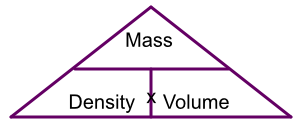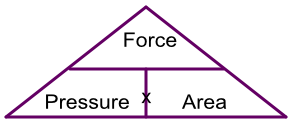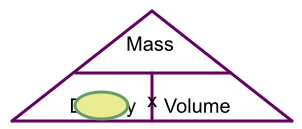Units of Density and Pressure

## Units of Density and Pressure

Density is the mass of a substance that occupies a given volume.

text(density) = frac(text(mass))(text(volume)), or D = frac(M)(V)

Density is normally measured using units of g/cm3 for smaller amounts, and kg/m3 for larger amounts.

Calculations of density can be represented by a triangle:To work out the mass, cover up mass on the triangle and you see density x volume. To work out density, cover up density and you see mass over volume, and for volume it is mass over density.

Pressure is a force acting on an area. A force pressing down on a point will exert more pressure than the same force acting over a wide area. Force is measured in Newtons (shown as N) and the area is normally given in square metres.

text(pressure) = frac(text(force))(text(area)), or P = frac(F)(A) and is shown as N/m2, or Nm-2

The formula can also be represented by a triangle:## Example 1

A pair of stiletto heels occupy an area of 2cm2. What is the pressure being applied to a floor if the weight of the woman wearing the stilettos is 560N?

Unknown is the pressure: using the triangle gives Force ÷ Area = 560 ÷ 2 = 280 N/cm2